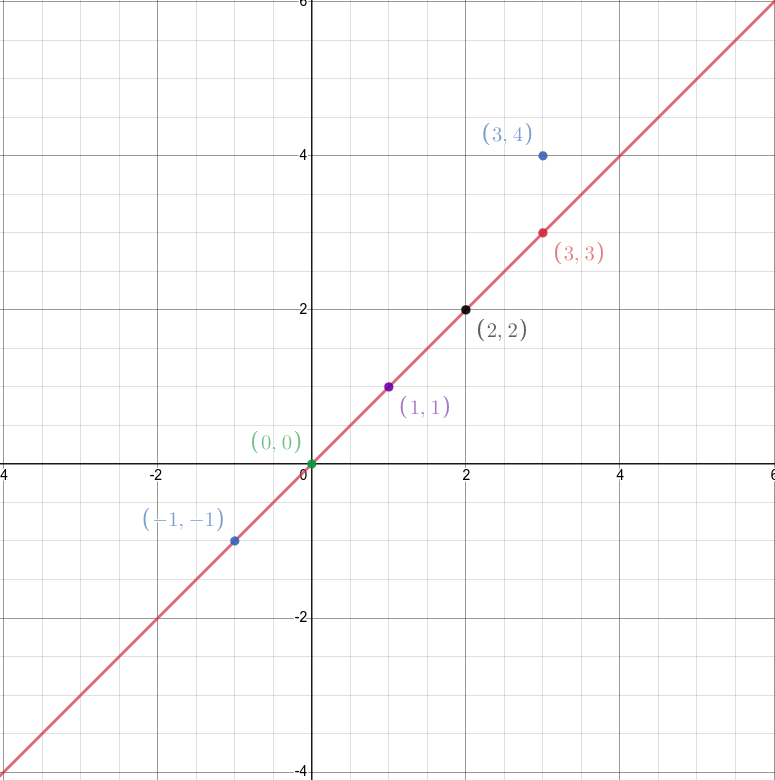7

# Maximum Points On Straight Line

Difficulty: HARDContributed By
Shrey Pansuria
Avg. time to solve
10 min
Success Rate
90%

Problem Statement
Suggest Edit

#### You are given a 2-D plane, and some 'N' integer coordinates in the form of (X, Y), where 'X' is the x-coordinate and 'Y' is the y-coordinate, all of which lie on that plane. You need to find the maximum number of coordinates among these which can form a straight line.

##### Note:
``````1. All the coordinates are integer coordinates.
2. There can be two identical coordinates.
``````
##### Input Format:
``````The first line of the input contains an integer 'T', denoting the number of test cases.

The first line of each test case contains the integer 'N', denoting the number of points.

The next N lines of each test case contain 2 space-separated integers which represent the coordinates of a given point.
``````
##### Output Format:
``````For each test case, every line of output prints the maximum number of points which lie on a straight line.
``````
##### Note :
``````You do not need to print anything, it has already been taken care of. Just implement the given function.
``````
##### Constraints:
``````1 <= T <=  50
1 <= N <= 10^3
-10^4 <= X, Y <= 10^4

Time Limit: 1 sec
``````
##### Sample Input 1:
``````2
2
1 1
5 5
6
-1 -1
0 0
1 1
2 2
3 3
3 4
``````
##### Sample Output 1:
``````2
5
``````
##### Explanation For Sample Output 1:
``````For the first test case, since there are only two points, they can always form  a straight line.

For the second test case, look at the picture below.
````````````We can easily see that 5 out of 6 points lie on a straight line. Hence 5 is the answer.
``````Console